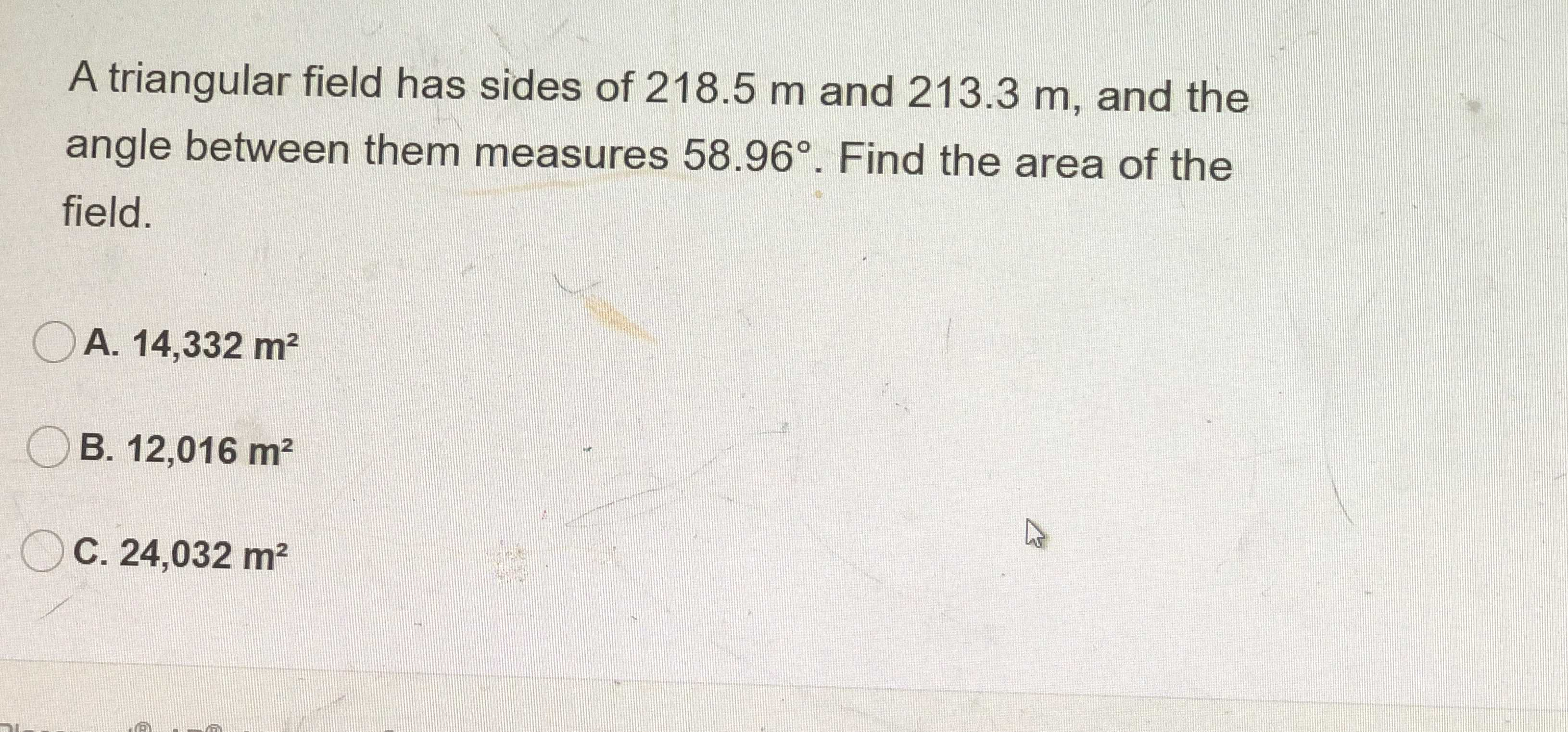### Still have math questions?

Trigonometry
QuestionA triangular field has sides of $$218.5 m$$ and $$213.3 m$$ , and the angle between them measures $$58.96 ^ { \circ }$$ . Find the area of the field.

A. $$14,332 m ^ { 2 }$$

B. $$12,016 m ^ { 2 }$$

C. $$24,032 m ^ { 2 }$$

$$A = 0.5\times side_ 1\times side_ 2\times \sin \theta \\ \theta is angle between them$$
$$Area = 0.5\times 218.5\times 213.3\times \sin { 58.96} = 19,966.207232 m^ 2$$